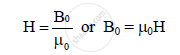Share

# Define Magnetic Intensity. - Physics

#### Questions

Define Magnetic intensity.

What is Magnetic intensity?

#### Solution 1

Magnetic intensity:- Magnetic intensity is a quantity used in describing magnetic phenomenon in terms of the magnetic field. The strength of the magnetic field at a point can be given in terms of a vector quantity called magnetic intensity (H).

#### Solution 2

Magnetic intensity: The ratio of the strength of magnetising field to the permeability of
free space is called as magnetic intensity.

The strength of magnetic field at a point can be given in terms of vector quantity called
as magnetic intensity (H).The SI unit of magnetic intensity is Am−1.

Is there an error in this question or solution?